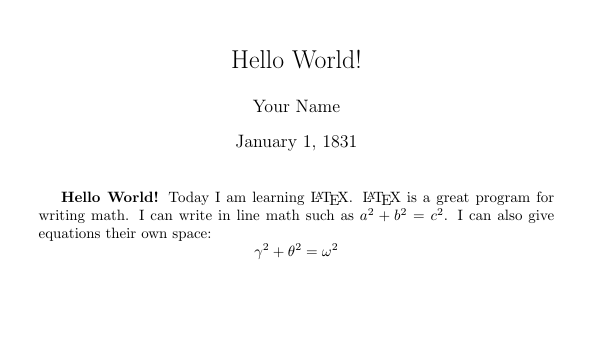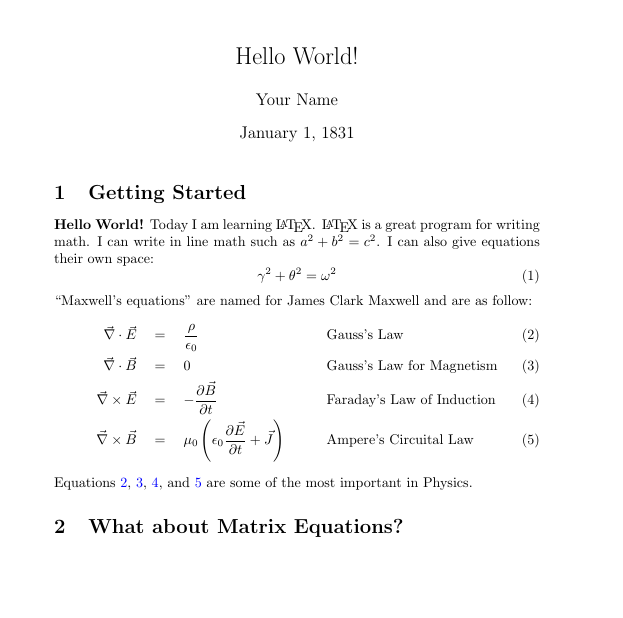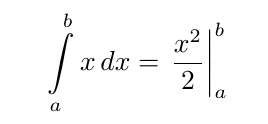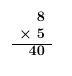It looks like you're using Internet Explorer 11 or older. This website works best with modern browsers such as the latest versions of Chrome, Firefox, Safari, and Edge. If you continue with this browser, you may see unexpected results.

# Quick Introduction to LaTeX: Exercises

A quick guide to install and start using LaTeX

## Introduction

The best way to learn LaTeX is by writing your own code and working through different situation.  In this section of the guide there are several samples problems, as well as their solutions.  The problems build off the previous ones and tend to get harder as you move along.  Feel free to reference back to the guide, use the hints. or use Google if you are stuck.  Remember there can be more than one way to answer some of these questions so if your solution doesn't match what is given but the code compiles correctly that is ok.

Keep practicing!

## Exercise 1: Hello World!

Create a document with the title Hello World!, your name, and todays date.  Include in the document the following text "Hello World!  Today I am learning LaTeX."  The solution should look like this (but with todays date):• Remember the first part of any document is the preamble and it must begin with \documentclass{} an article is a good document class for this exercise.
• To make the title look nice use the command \maketitle, but make sure you put it in the body of the document
• For \maketitle to work correctly you will want to use \title{}, \author{}, and \date{}, but they don't belong in the document body.
• What happens if you don't include \date{}? What happens if you use \date{} with no argument? What if you use \date{\today}?
• To start the body of your document use the command \begin{document}.  To end your document use the command \end{document}
• Try the command \LaTeX

\documentclass{article}

\title{Hello World!}
\date{January 1, 1831}
\begin{document}
\maketitle

\textbf{Hello World!} Today I am learning \LaTeX.
\end{document}

## Exercise 2: Adding some simple math

Add to the document you created in exercise 1 the following text:

"LaTeX is a great program for writing math.  I can write in line math such as a^2 + b^2 = c^2.   I can also give equations their own space: gamma^2 +theta^2 = omega^2"

Your final document should look like this:• Is there a problem with the spacing after the second \LaTeX?  Try using \LaTeX{} an empty argument can improve spacing with some commands.
• To write in line math mode you need to include a before and after the text that should be written as math. • before and after an equation or $and$ will cause LaTeX to entire a display environment • If you don't know how to create Greek letters check out the resources tab. • Use ^ for superscripts. Use _ for subscripts. \documentclass{article} \title{Hello World!} \author{Your Name} \date{January 1, 1831} \begin{document} \maketitle \textbf{Hello World!} Today I am learning \LaTeX. \LaTeX{} is a great program for writing math. I can write in line math such as a^2+b^2=c^2. I can also give equations their own space: $\gamma^2+\theta^2=\omega^2$ \end{document} ## Exercise 3: More Difficult Math In this exercise you will build onto the document created in exercises 1 and 2 with more complicated math and structures. This is a much more difficult exercise so take your time. First give the display environment equation from exercise 2 an equation number. Second add the following line of text (make sure there it is not indented as a new paragraph: "Maxwell's equations" are named for James Clark Maxwell and are as follow: Now write Maxwell's equations (as seen below), use an align environment and align the equations at the = signs and at the equations names.• Did you include the amsmath package command, \usepackage{amsmath}? Some symbols and advanced math environments such as align will not work with out it! • The equation environment, \begin{equation}, automatically puts you in display mode and includes equations numbers. If you want to use this mode but don't want equation numbers use equation* • To create quotation marks in LaTeX use the symbol  (the ~ key) twice on the left and use the ' key twice on the right. Using " on the left and right will not work properly. • In the align environment you use the & to denote points of alignment. If you want a second alignment point use &&. • If you are having trouble with spacing use around = use the command \quad this adds extra horizontal space. • When adding text in math mode you need to use the command \text{} where the text you want added is the argument. This command tells LaTeX to use regular text at that location. • \vec{} will create vector notation. \partial will give you partial derivatives, and remember for fractions use \frac{numerator}{denomonator}. • If you are having trouble getting your parenthesis to look right use the command \left( and \right) this will automatically fit the parenthesis to the equation within. This also works with \left[\left{, and \left|, but you always need a \right to go with it. \documentclass{article} \usepackage{amsmath} \title{Hello World!} \author{Your Name} \date{January 1, 1831} \begin{document} \maketitle \textbf{Hello World!} Today I am learning \LaTeX. \LaTeX{} is a great program for writing math. I can write in line math such as a^2+b^2=c^2. I can also give equations their own space: \begin{equation} \gamma^2+\theta^2=\omega^2 \end{equation} Maxwell's equations'' are named for James Clark Maxwell and are as follow: \begin{align} \vec{\nabla} \cdot \vec{E} \quad &=\quad\frac{\rho}{\epsilon_0} &&\text{Gauss's Law} \\ \vec{\nabla} \cdot \vec{B} \quad &=\quad 0 &&\text{Gauss's Law for Magnetism}\\ \vec{\nabla} \times \vec{E} \quad &=\hspace{10pt}-\frac{\partial{\vec{B}}}{\partial{t}} &&\text{Faraday's Law of Induction} \\ \vec{\nabla} \times \vec{B} \quad &=\quad \mu_0\left( \epsilon_0\frac{\partial{\vec{E}}}{\partial{t}}+\vec{J}\right) &&\text{Ampere's Circuital Law} \end{align} \end{document} ## Exercise 4: Creating Sections and Referencing Equation Labels and reference are very simple to execute in LaTeX, can be used with any numbered object such as figures, equations, and sections, and are automatically updated whenever the document is complied. If, for example, you realized you forgot an equation somewhere in the middle of your document, between 10 other equations, all the equations after the newly inserted equation would automatically be renumbered and proper references to them will reflect this new numbering! For this exercise take the document made in the previous exercise and create two sections one at the beginning (Getting Started) and one after all your texts (What about Matrix Equations?). Also add to your document references to each of Maxwell's Equations, and then write a sentence that references each equation. You will need to use the commands \label{} and \ref{}. It is common practice in LaTeX when labeling to use the format eq:name, fig:name, tab:name, and so on depending on the type of object you are labeling. If you are confused by this look at the labels in the solution Also, if you would like your citations to act as hyperlinks you need to use the package hyperref\usepackage{hyperref}, remember with this package you can change the default hyperlink settings with the command \hypersetup{} in the preamble i.e. \hypersetup{colorlinks=true, linkcolor=blue, urlcolor=blue, citecolor=blue}.• For sections use the \section{} command. • Make sure you use the \label{} command at the end of each equation before the line break command \\. • You may need compile your document twice in order to get cross references to work. \documentclass{article} \usepackage{amsmath} \usepackage{hyperref} \hypersetup{colorlinks=true, linkcolor=blue, ur lcolor=blue, citecolor=blue} \title{Hello World!} \author{Your Name} \date{January 1, 1831} \begin{document} \maketitle \section{Getting Started} \textbf{Hello World!} Today I am learning \LaTeX. \LaTeX{} is a great program for writing math. I can write in line math such as a^2+b^2=c^2. I can also give equations their own space: \begin{equation} \gamma^2+\theta^2=\omega^2 \end{equation} Maxwell's equations'' are named for James Clark Maxwell and are as follow: \begin{align} \vec{\nabla} \cdot \vec{E} \quad &=\quad\frac{\rho}{\epsilon_0} &&\text{Gauss's Law} \label{eq:GL}\\ \vec{\nabla} \cdot \vec{B} \quad &=\quad 0 &&\text{Gauss's Law for Magnetism} \label{eq:GLM}\\ \vec{\nabla} \times \vec{E} \quad &=\hspace{10pt}-\frac{\partial{\vec{B}}}{\partial{t}} &&\text{Faraday's Law of Induction} \label{eq:FL}\\ \vec{\nabla} \times \vec{B} \quad &=\quad \mu_0\left( \epsilon_0\frac{\partial{\vec{E}}}{\partial{t}}+\vec{J}\right) &&\text{Ampere's Circuital Law} \label{eq:ACL} \end{align} Equations \ref{eq:GL}, \ref{eq:GLM}, \ref{eq:FL}, and \ref{eq:ACL} are some of the most important in Physics. \section{What about Matrix Equations?} \end{document} ## Exercise 5: Creating Matrix Equations In this exercise add the matrix equation to your document as seen below:• There are different matrix environments in LaTeX such as matrix, pmatrix, and bmatrix. • In a matrix use the & character to denote new columns and the \\ command to start new rows. • If you are having trouble creating the dots use the commands \dots, \ddots, and \vdots \documentclass{article} \usepackage{amsmath} \usepackage{hyperref} \hypersetup{colorlinks=true, linkcolor=blue, urlcolor=blue, citecolor=blue} \title{Hello World!} \author{Your Name} \date{January 1, 1831} \begin{document} \maketitle \section{Getting Started} \textbf{Hello World!} Today I am learning \LaTeX. \LaTeX{} is a great program for writing math. I can write in line math such as a^2+b^2=c^2. I can also give equations their own space: \begin{equation} \gamma^2+\theta^2=\omega^2 \end{equation} Maxwell's equations'' are named for James Clark Maxwell and are as follow: \begin{align} \vec{\nabla} \cdot \vec{E} \quad &=\quad\frac{\rho}{\epsilon_0} &&\text{Gauss's Law} \label{eq:GL}\\ \vec{\nabla} \cdot \vec{B} \quad &=\quad 0 &&\text{Gauss's Law for Magnetism} \label{eq:GLM}\\ \vec{\nabla} \times \vec{E} \quad &=\hspace{10pt}-\frac{\partial{\vec{B}}}{\partial{t}} &&\text{Faraday's Law of Induction} \label{eq:FL}\\ \vec{\nabla} \times \vec{B} \quad &=\quad \mu_0\left( \epsilon_0\frac{\partial{\vec{E}}}{\partial{t}}+\vec{J}\right) &&\text{Ampere's Circuital Law} \label{eq:ACL} \end{align} Equations \ref{eq:GL}, \ref{eq:GLM}, \ref{eq:FL}, and \ref{eq:ACL} are some of the most important in Physics. \section{What about Matrix Equations?} \begin{equation*} \begin{pmatrix} a_{11}&a_{12}&\dots&a_{1n}\\ a_{21}&a_{22}&\dots&a_{2n}\\ \vdots&\vdots&\ddots&\vdots\\ a_{n1}&a_{n2}&\dots&a_{nn} \end{pmatrix} \begin{bmatrix} v_{1}\\ v_{2}\\ \vdots\\ v_{n} \end{bmatrix} = \begin{matrix} w_{1}\\ w_{2}\\ \vdots\\ w_{n} \end{matrix} \end{equation*} \end{document} ## Exercise 6: Tables and Figures For this exercise create a new section to your document for figures and tables. In that section create a table and a figure. Give each object a caption, try putting the table caption above the table and the figure caption below. Try this with a figure of your choice.• To include a caption with your table use the environment table, \begin{table}. • To create the table itself you need to use the environment tabular, \begin{tabular}{}. • In the second argument of tabular you will define the number of columns in the table, the justification of each column, as well as if you would like any lines between the columns. In the table used in this question the command \begin{tabular}{|l||c|c|r|} was used. This creates a 4 column matrix, the first column is left justified, l, the middle two are center justified, c, and the last column is right justified, r. The table also has borders on the outside as well as between the columns using the | (shift backslash key). Notice there is a double || after the first column. • Entering values into your table works much like a matrix. use the & character to separate columns and the \\ command to start working on a new row. Use if you want to write in math mode. • If you want to create a horizontal line at the top, bottom, or between rows of your table use the command \hline at the start of the row and after the last \\. • Placing the command \centering at the start of the table of figure environment will center the object. • For figures you need to include the graphicx package, \usepackage{graphicx}. • Using the graphicx package you can create an environment figure, \begin{figure}. • To insert your figure use the command \includegraphics[]{}. • The optional argument of \includegraphics can be used to resize the figure try [width=\textwidth] and [width=.5\textwidth] and see the difference it makes. • The required argument of \includegraphics is the name of the file, DO NOT include the file type and make sure the file is located in the same folder as the LaTeX document otherwise it is more complicated to include it. For example the argument for file bern.jpg should just be \includegraphics{bern}. • When including a floating object in your document such as a figure or a table you can follow the required argument with an additional optional argument to indicate the preferred placement of the object. The letter h indicates here (current location), b indicates bottom (bottom of a page), and t indicated top (top of page). Using an ! stresses to LaTeX to make this placement. So you may see commands for figures of tables that look like this \begin{figure}[hbt!]. \documentclass{article} \usepackage{amsmath} \usepackage{hyperref} \hypersetup{colorlinks=true, linkcolor=blue, urlcolor=blue, citecolor=blue} \usepackage{graphicx} \title{Hello World!} \author{Your Name} \date{January 1, 1831} \begin{document} \maketitle \section{Getting Started} \textbf{Hello World!} Today I am learning \LaTeX. \LaTeX{} is a great program for writing math. I can write in line math such as a^2+b^2=c^2. I can also give equations their own space: \begin{equation} \gamma^2+\theta^2=\omega^2 \end{equation} Maxwell's equations'' are named for James Clark Maxwell and are as follow: \begin{align} \vec{\nabla} \cdot \vec{E} \quad &=\quad\frac{\rho}{\epsilon_0} &&\text{Gauss's Law} \label{eq:GL}\\ \vec{\nabla} \cdot \vec{B} \quad &=\quad 0 &&\text{Gauss's Law for Magnetism} \label{eq:GLM}\\ \vec{\nabla} \times \vec{E} \quad &=\hspace{10pt}-\frac{\partial{\vec{B}}}{\partial{t}} &&\text{Faraday's Law of Induction} \label{eq:FL}\\ \vec{\nabla} \times \vec{B} \quad &=\quad \mu_0\left( \epsilon_0\frac{\partial{\vec{E}}}{\partial{t}}+\vec{J}\right) &&\text{Ampere's Circuital Law} \label{eq:ACL} \end{align} Equations \ref{eq:GL}, \ref{eq:GLM}, \ref{eq:FL}, and \ref{eq:ACL} are some of the most important in Physics. \section{What about Matrix Equations?} \begin{equation*} \begin{pmatrix} a_{11}&a_{12}&\dots&a_{1n}\\ a_{21}&a_{22}&\dots&a_{2n}\\ \vdots&\vdots&\ddots&\vdots\\ a_{n1}&a_{n2}&\dots&a_{nn} \end{pmatrix} \begin{bmatrix} v_{1}\\ v_{2}\\ \vdots\\ v_{n} \end{bmatrix} = \begin{matrix} w_{1}\\ w_{2}\\ \vdots\\ w_{n} \end{matrix} \end{equation*} \section{Tables and Figures} Creating a Table is not unlike creating a matrix: \begin{table}[hbt!] \centering \caption{This is a table that shows how to create different lines as well as different justifications} \begin{tabular}{|l||c|c|r|} \hline x&1&2&3\\ \hline f(x)&4&8&12\\ f(x)&4&8&12\\ \hline \end{tabular} \end{table} \begin{figure} \centering \includegraphics[width=\textwidth]{bern} \caption{Bern Dibner Library} \end{figure} \end{document} ## Exercise 7: Bibliography Before attempting this exercise make sure you have read through the BibTex tab of this guide. Create a BibTex file with a few citations in it. Add a sentence that contains these citations and then add a bibliography to your document.• It is much easier to create a BibTex file using a citation management tool like Zotero or Mendeley. • Use the \cite{} command where the argument is bibID for the citation in the .bib file. • If you are citing multiple items at the same location you can use \cite{} command and separate the bibIDs with commas. • Make sure use the command \bibliographystyle{} to tell LaTeX which bibliographic style to use. • Some common styles are plain, ieeetr, acm, and apalike. • To create your bibliography use the command \biblography{} where the argument is the name of the bib file. DO NOT include .bib and MAKE SURE the .bib file is located in the same folder as the LaTeX document. • You may need to compile your document twice in order to get cross references to work correctly \documentclass{article} \usepackage{amsmath} \usepackage{hyperref} \hypersetup{colorlinks=true, linkcolor=blue, urlcolor=blue, citecolor=blue} \usepackage{graphicx} \title{Hello World!} \author{Your Name} \date{January 1, 1831} \begin{document} \maketitle \section{Getting Started} \textbf{Hello World!} Today I am learning \LaTeX. \LaTeX{} is a great program for writing math. I can write in line math such as a^2+b^2=c^2. I can also give equations their own space: \begin{equation} \gamma^2+\theta^2=\omega^2 \end{equation} `Maxwell's equations'' are named for James Clark Maxwell and are as follow: \begin{align} \vec{\nabla} \cdot \vec{E} \quad &=\quad\frac{\rho}{\epsilon_0} &&\text{Gauss's Law} \label{eq:GL}\\ \vec{\nabla} \cdot \vec{B} \quad &=\quad 0 &&\text{Gauss's Law for Magnetism} \label{eq:GLM}\\ \vec{\nabla} \times \vec{E} \quad &=\hspace{10pt}-\frac{\partial{\vec{B}}}{\partial{t}} &&\text{Faraday's Law of Induction} \label{eq:FL}\\ \vec{\nabla} \times \vec{B} \quad &=\quad \mu_0\left( \epsilon_0\frac{\partial{\vec{E}}}{\partial{t}}+\vec{J}\right) &&\text{Ampere's Circuital Law} \label{eq:ACL} \end{align} Equations \ref{eq:GL}, \ref{eq:GLM}, \ref{eq:FL}, and \ref{eq:ACL} are some of the most important in Physics. \section{What about Matrix Equations?} \begin{equation*} \begin{pmatrix} a_{11}&a_{12}&\dots&a_{1n}\\ a_{21}&a_{22}&\dots&a_{2n}\\ \vdots&\vdots&\ddots&\vdots\\ a_{n1}&a_{n2}&\dots&a_{nn} \end{pmatrix} \begin{bmatrix} v_{1}\\ v_{2}\\ \vdots\\ v_{n} \end{bmatrix} = \begin{matrix} w_{1}\\ w_{2}\\ \vdots\\ w_{n} \end{matrix} \end{equation*} \section{Tables and Figures} Creating a Table is not unlike creating a matrix: \begin{table}[h!] \centering \caption{This is a table that shows how to create different lines as well as different justifications} \begin{tabular}{|l||c|c|r|} \hline x&1&2&3\\ \hline f(x)&4&8&12\\ f(x)&4&8&12\\ \hline \end{tabular} \end{table} \begin{figure}[h!] \centering \includegraphics[width=\textwidth]{bern} \caption{Bern Dibner Library} \end{figure} \section{Bibliography} You will probably want references in your document so that you can cite articles like \cite{frenkel_fine_2013, frenkel_optical_2013, frenkel_temperature_2012, frenkel_whispering-gallery_2013,frenkel_-chip_2016} \bibliographystyle{ieeetr} \bibliography{bibl} \end{document} The following is the BibTex file bibl.bib created using Zotero: @inproceedings{frenkel_fine_2013, title = {Fine temperature measurement and fabrication of on-chip whispering-gallery mode micro-sensors}, url = {http://proxy.library.nyu.edu/login?url=http://search.ebscohost.com/login.aspx?direct=true&db=edselc&AN=edselc.2-52.0-84901804547&site=eds-live}, doi = {10.1115/MNHMT2013-22003}, booktitle = {{ASME} 2013 4th {International} {Conference} on {Micro}/{Nanoscale} {Heat} and {Mass} {Transfer}, {MNHMT} 2013}, publisher = {American Society of Mechanical Engineers (ASME)}, author = {Frenkel, M. and Avellan, M. and Guo, Z.}, year = {2013}, note = {Conference Proceedings} } @inproceedings{frenkel_optical_2013, title = {Optical whispering-gallery mode phenomenon as a composite sensor with applications to direct on-chip thermal sensing}, volume = {4}, isbn = {978-0-7918-5550-8}, url = {http://proxy.library.nyu.edu/login?url=http://search.ebscohost.com/login.aspx?direct=true&db=edselc&AN=edselc.2-52.0-84893001861&site=eds-live}, doi = {10.1115/HT2013-17245}, booktitle = {{ASME} 2013 {Heat} {Transfer} {Summer} {Conf}.{Collocated} with the {ASME} 2013 7th {Int}.{Conf}.on {Energy} {Sustainability} and the {ASME} 2013 11th {Int}.{Conf}.on {Fuel} {Cell} {Science}, {Engineering} and {Technology}, {HT} 2013}, author = {Frenkel, M. and Avellan, M. and Guo, Z.}, year = {2013}, note = {Conference Proceedings} } @inproceedings{frenkel_temperature_2012, title = {Temperature sensing of joule heating inside an optical whispering-gallery mode micro-annulus}, volume = {2}, isbn = {978-0-7918-4478-6}, url = {http://proxy.library.nyu.edu/login?url=http://search.ebscohost.com/login.aspx?direct=true&db=edselc&AN=edselc.2-52.0-84892648569&site=eds-live}, doi = {10.1115/HT2012-58133}, booktitle = {{ASME} 2012 {Heat} {Transfer} {Summer} {Conf}.{Collocated} with the {ASME} 2012 {Fluids} {Engineering} {Div}.{Summer} {Meeting} and the {ASME} 2012 10th {Int}.{Conf}.on {Nanochannels}, {Microchannels} and {Minichannels}, {HT} 2012}, author = {Frenkel, M. and Avellan, M. and Guo, Z.}, year = {2012}, note = {Conference Proceedings}, pages = {823--826} } @article{frenkel_whispering-gallery_2013, title = {Whispering-gallery mode composite sensors for on-chip dynamic temperature monitoring}, volume = {24}, issn = {09570233}, url = {http://proxy.library.nyu.edu/login?url=http://search.ebscohost.com/login.aspx?direct=true&db=edswsc&AN=000320449100036&site=eds-live}, abstract = {Whispering-gallery mode temperature microsensors have been demonstrated to have extremely high accuracy. Previous experiments have been limited to indirect sensor heating by externally heating the local environment. In this paper, we coated PDMS films directly onto an electrical resistive wire as sensors, allowing on-chip dynamic temperature measurement. The effects of sensor size are discussed and verified through an expansion of the current theory of WGM resonance shifts to include composite materials. Finally, the WGM sensor's measurements are compared to the same measurements recorded by a thermocouple, demonstrating the great advantages of WGM sensors for on-chip real temperature monitoring.}, number = {7}, journal = {MEASUREMENT SCIENCE AND TECHNOLOGY}, author = {Frenkel, M. and Avellan, M. and Guo, Z. X.}, year = {2013}, keywords = {ENGINEERING, MULTIDISCIPLINARY, INSTRUMENTS \& INSTRUMENTATION, on-chip measurement, optical sensor, temperature measurement} } @article{frenkel_-chip_2016, title = {On-chip, dynamic, and cryogenic temperature monitoring via {PDMS} micro-bead coatings}, volume = {54}, issn = {1099-0488}, url = {http://dx.doi.org/10.1002/polb.24016}, doi = {10.1002/polb.24016}, abstract = {Polydimethylsiloxane (PDMS) microshells/beads coated onto an electrical current-carrying wire are demonstrated for on-chip, dynamic, cryogenic temperature measurement via monitoring optical whispering-gallery mode (WGM) frequency shifts. PDMS is found to be capable of supporting WGM resonance at cryogenic temperatures down to 95 K, limited by the present lab-built cryogenic working environment. The effect of the polymeric sensor diameter on temperature sensitivity is explored and discussed. The sensors are tested for their real-time temperature monitoring capabilities and accuracy in the cryogenic temperature regime of 95–140 K, and a comparison to a theoretical model, where the electrical resistivity of nichrome wire at cryogenic temperature is also experimentally determined, is examined. © 2016 Wiley Periodicals, Inc. J. Polym. Sci., Part B: Polym. Phys. 2016, 54, 1118–1124}, number = {12}, journal = {Journal of Polymer Science Part B: Polymer Physics}, author = {Frenkel, Matthew and Guo, Zhixiong}, year = {2016}, keywords = {coatings, cryogenic temperature, electrical resistivity, films, optical resonance, sensors}, pages = {1118--1124} } ## Additional Exercises: More Equations A) Trying creating the following calculus equation:B) If you are feeling really good try your luck with these equations (no hints are given)Hints for part A only • To get the spacing right try using the command \, before dx on the left side of the equation • The command \limits helps position the integral limits where you want them, \limits_a^b. • To get the size of the line at the end of the equation try using the command \right| • To use this equation without a command \left| creating lines on both sides of the equation place the command \left. on the left side of the equation. This technique can be used with any of the \right or \left commands if you only want an object on one side of your equation. Part A $\int\limits_a^b x\,dx = \left.\frac{x^2}{2} \right|_a^b$ Part B \begin{equation} \iiint\limits_V f(x,y,z)\,dV = F \end{equation} \begin{equation} \frac{dx}{dy}=x'=\lim_{h \to 0}\frac{f(x+h)-f(x)}{h} \end{equation} \begin{equation} |x|=\begin{cases} -x, & \text{if x < 0}\\ x, & \text{if x \geq 0} \end{cases} \end{equation} \begin{equation} F(x)= A_0 + \sum_{n=1}^N\left[ A_n\cos{\left(\frac{2\pi nx}{P}\right)}+B_n\sin{\left(\frac{2\pi nx}{P}\right)}\right] \end{equation} \begin{equation} \sum_n \frac{1}{n^s}=\prod_p \frac{1}{1-\frac{1}{p^s}} \end{equation} \begin{equation*} % equation* suppress equation numbering same for align* m\ddot{x}+c\dot{x}+kx=F_0\sin(2\pi ft) \end{equation*} \begin{align*} f(x)\quad &=\quad x^2 + 3x + 5x^2 +8 +6x\\ &=\quad 6x^2 +9x +8\\ &=\quad x(6x+9)+8 \end{align*} X=\frac{F_0}{k}\frac{1}{\sqrt{(1-r^2)^2+(2\zeta r)^2}} $$\begin{equation} G_{\mu\nu} \equiv R_{\mu\nu}-\frac{1}{2}Rg_{\mu\nu}=\frac{8\pi G}{c^4}T_{\mu\nu} \end{equation}\\$$\mathrm{6CO_2+6H_2O \to C_6H_{12}O_6+6O_2}\mathrm{SO_4^{2-}+Ba^{2+} \to BaSO_4 }\begin{equation} \begin{pmatrix} a_{11}&a_{12}&\dots&a_{1n}\\ a_{21}&a_{22}&\dots&a_{2n}\\ \vdots&\vdots&\ddots&\vdots\\ a_{n1}&a_{n2}&\dots&a_{nn} \end{pmatrix} \begin{pmatrix} v_{1}\\ v_{2}\\ \vdots\\ v_{n} \end{pmatrix} = \begin{pmatrix} w_{1}\\ w_{2}\\ \vdots\\ w_{n} \end{pmatrix} \end{equation} \begin{equation} \frac{\partial{\bf{u}}}{\partial{t}}+(\bf{u}\cdot\nabla)\bf{u}-\nu\nabla^2\bf(u)=-\nabla h \end{equation} $% This is preferred to the environment \alpha A \beta B \gamma \Gamma \delta \Delta \pi \Pi \omega \Omega$ ## Additional Exercises: \newcommand In this exercise try your hand at creating a new command: A) Create a command that will display a multiplication problem in the pictured format if you give it the two numbers to be multipliedB) Can you adapt to code so that it will also display the answer, if providedC) Can you code several rows of problems?• You will need to use \newcommand{new command name}{what command does} • use the \ key when inputting the new command name into the \newcommand • Tell new command how many inputs to expect with an optional command. \newcommand{name}{what command does} • As a placeholder for the arguments of your new command use the # and the number of that command. For example \newcommand{\largeandbold}{\Large{\textbf{#1}}} will create a command called largeandbold that will increase the size and use boldface for a single argument. • Try using a \tabular in your new command if you are having trouble with alignment • \times gives you the nice multiplication symbol Part A \documentclass[11pt]{article} \usepackage{amsmath} \newcommand{\mul}[]{ \quad\large{ \begin{tabular}{r} \textbf{#2}\\\boldsymbol{\times}$\textbf{#1}\\ \hline \vspace{3pt}\\ \vspace{10pt}\\ \end{tabular} }} \begin{document} \mul{5}{8} \end{document} Part B Part A \documentclass[11pt]{article} \usepackage{amsmath} \newcommand{\mul}[]{ \quad\large{ \begin{tabular}{r} \textbf{#3}\\$\boldsymbol{\times}$\textbf{#2}\\ \hline \vspace{3pt} \textbf{#1}\\ \vspace{10pt}\\ \end{tabular} }} \begin{document} \mul{5}{8} \end{document} Part C \documentclass[11pt]{article} \usepackage{amsmath} \newcommand{\mul}[]{ \quad\large{ \begin{tabular}{r} \textbf{#3}\\$\boldsymbol{\times}\$ \textbf{#2}\\
\hline \vspace{3pt}
\textbf{#1}\\
\vspace{10pt}\\
\end{tabular}
}}

\begin{document}

\begin{tabular}{ccccccc}

\mul{5}{8} & \mul{4}{12} &\mul{3}{2}& \mul{10}{7} &\mul{5}{5}& \mul{6}{4}& \mul{8}{9}\\
\mul{12}{12}&  \mul{4}{1}& \mul{9}{6}& \mul{7}{5} &\mul{5}{11}& \mul{8}{8}& \mul{7}{12}\\
\mul{5}{1}&  \mul{3}{4}& \mul{6}{2}& \mul{5}{5}& \mul{9}{3}& \mul{1}{12}& \mul{0}{8}\\

\end{tabular}

\end{document}